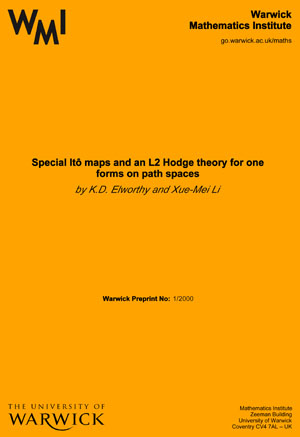# WMI Preprints 1975

Characteristic classes of foliations,
H.V. Pittie

A clock and wavefront model for control of the number for repeated structures during animal morphogenesis,
by J. Cooke & E.C. Zeeman

Compact deformations on conformally flat structures on almost symplectic Banach-manifolds,
by H. Haahti

Conjugacy theorems for a class of locally finite Lie algebras,
by I. Stewart

Unsmoothable diffeomorphisms,
by J. Harrison

Tensor products, operator ideals and functors on categories of Banach spaces,
by P. Michor

A cyclinder flow arising from irregularity of distribution,
by K. Schmidt

Transversality in G-manifolds (part 1),
by M. Field

Central limit theorems for positive definite functions on Lie groups,
by K.R. Parthasarathy

A new method for constructing factorisable representations for current groups and current algebras,
by K.R. Parthasarathy & K. Schmidt

Infinite invariant measures on the circle,
by K. Schmidt

Topological classification of endomorphisms of line bundles,
by D.W. Bass

Centralizers of semi-simple elements in finite Chevalley groups,
by R.W. Carter

Duality of compactological and locally compact groups,
by J.B. Cooper & P. Michor

On the Green polynomials of classical groups,
by G. Lusztig

On the cohomology of a hyperfinite action,
by K.R. Parthasarathy & K. Schmidt

Finite groups which admit a fixed-point-free automorphism of order rst,
by P.J. Rowley

Every ergodic transformation has nonatomic invariant measures,
by K. Schmidt

Transversality in G-manifolds (part 2),
by M. Field

A Fitting class construction,
by T.O. Hawkes

On Radon-Nikodym derivatives of quasi-invariant measures,
by K. Schmidt

The use of directed graphs in the enumeration of orthogonal space groups,
by R.L.E. Schwarzenberger

La théorie des points fixes des applications à itérée condensante,
by J. Eells & G. Fournier

Nonlinear structures determined by measures on Banach spaces,
by K.D. Elworthy

A finitary classification of topological Markov chains and sofic systems,
by W. Parry

Uncountable Artinian modules and uncountable unsoluble groups satisfying min-n,

by B. Hartley

Transversality in G-manifolds and an equivariant version of Thom's transversality theorem,
by M.J. Field

A conjecture of Bachmuth and Mochizuki on automorphisms of soluble groups,
by B. Hartley

Thom's classification theorem (an intuitive approach),
by T. Poston & I. Stewart

### Search WMI Preprint Catalogue: